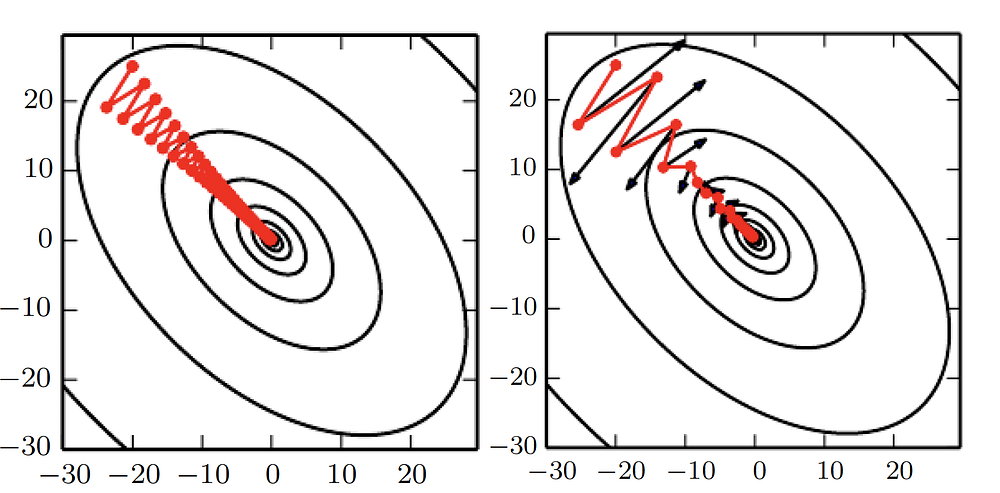# [DL] 7. Optimization Techniques on Gradient Descent and Learning Rate

Original article can be found here (source): Deep Learning on Medium# 1. Recap

## (1) Learning vs Optimization

Optimization is simply to reduce the training error whereas, the learning is to reduce the generalization error while keeping the training error small.

## (3) Mini-batch

The empirical risk is defined over the entire training set of m samples. But in most cases, we do not use the whole dataset to make single weight-update and, therefore, we do not compute this expected value exactly. Furthermore, computing empirical risk over the whole dataset can be severally expensive to evaluate the model as we have millions of training images in modern vision datasets.

As a result, using a subset of the dataset which is a technique called mini-batch to evaluate the model and to update the parameters(weights) is more commonly used. In the majority of cases, the learning algorithms converge faster with this way since we use the rapid approximation of gradients, not the slower exact gradients.

The cost function of neural networks with many layers and non-linear activations often have very steep regions in the parameter(weight) space. Such cliffs and sharp regions are the result of multiplying large weights together from many layers.

The problem of those cliffs happens when the parameters(weights) get close to such sharp regions as they return significantly large gradients. Because of large gradients, the updated parameter might go unexpectedly far away from the minima since the step-size of parameter-update made by the gradient descent algorithm depends on the norm of gradient with respect to such a parameter. This often cause the loss of the previous optimization works on parameters.

• How to solve the gradient exploding issue?

This issue can be resolved using the gradient clipping. It is simply not allowing the weight update which is larger than the predetermined threshold. More intuitively, the gradient clipping caps the step on the parameter space to prevent weights from moving too far away.

## (6) Learning Rate

The learning rate 𝛜 is one of the most crucial hyperparameters of the neural networks. It defines how large or small the step-size in the parameter space is. The larger the learning rate is, the farther parameters get updated. In practice, it is common to decay the learning rate over iteration.

This is reasonable since in the early stage of the learning, the weights are likely to be far away from the minima therefore, large step-size is desirable. Whereas, in the end phase of learning the weights are already close to the minima. So in this case, making small updates on weights(parameter) would increase the chance of reaching the minim. We will deal with some techniques that how we change the learning rate over the iteration at the later part of this chapter.

Figure 5 illustrates the behavior of loss function over the epoch by the different values of the learning rate. With an appropriately set learning rate, it shows a descending trend which has a dramatic decrease in the first few epochs and then slowly converges at the end phase of the training.

# 2. SGD with momentum

SGD is great but it often is not as enough fast as we want. In order for SGD to boost the training, we can apply the concept of momentum.

The momentum 𝛎 accumulates the gradients from the first iteration until the current step with exponential decay term 𝛂. What this accumulation in 𝛎 is that if the calculated directional derivative g in current iteration has the same direction as 𝛎 then we make the weight-update in the same direction as g but with the larger size of step, meaning that we update weights with bigger values in parameter space compared to when we update weights without momentum 𝛎. Therefore, eventually, the SGD reaches the destination faster with momentum 𝛎 than when it is without 𝛎.

Let’s take a closer look at its weight-update rule and graphs.